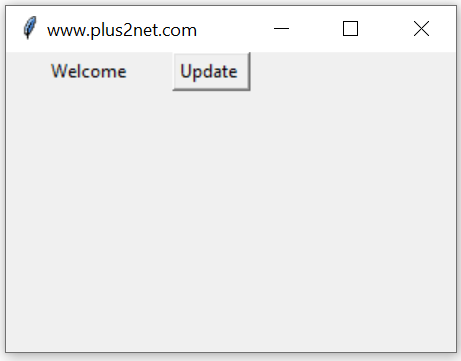# Python tkinter IntVar() trace

``r_v=tk.IntVar(master,value,name)``
`master`: (Optional)The variable is associated with, default value is parent window.
`value`:(Optional) We can set the initial value for the variable.
`name` : (Optional) Name given default is PY_VAR1

Tkitner IntVar() get(), set(), trace() methods to manage data and trigger call back functions

For an IntVar() so we can check the different modes of this variable, like this

w :Write- the variable is written by someone.
u :undefined – The variable is deletedHere is an example which uses w ( write ) mode to display the value of the variable when ever it changes.
We used one Button and used on Click event to change the value of this variable db1 from 5 to 10.
``b1 = tk.Button(my_w,text='Update',command=lambda:db1.set(10))``
This triggers the trace() which used the callback function my_r() to print the value of the variable ( 10 ) to our console.
``````import tkinter as tk
from tkinter import *
my_w = tk.Tk()
my_w.geometry("300x100")  # Size of the window

def my_r(*args):
print(int1.get()) #  Print when variable changes.

int1 = tk.IntVar(my_w) # declare IntVar()
int1.set(5)

b1 = tk.Button(my_w,text='Update',command=lambda:int1.set(10))

int1.trace('w',my_r) # monitor the change of variable
my_w.mainloop()``````
Output
``10``
Use StringVar() for handling String data
Use DoubleVar() for handling Float Data

## set() and get() methods of IntVar

In above examples we used set() method to assign data to the Integer variable and to read the data stored we used get() method. These two methods are frequently used in our scripts.
``````int1.set(10) # assign value to int1
print(int1.get()) # display the value assigned to int1``````

## Initializing IntVar

We can using set() method to assign data to IntVar, after declaring or we can assign value while declaring the string variable.
``int1=tk.IntVar(value=5) # Assign value to int1``

## Length of IntVar

Before using len function, we have to convert the IntVar to string by using str()
``print(len(str(int1.get())))``

## Normal Variable and IntVar

IntVar() is a class in Tkinter. In a GUI application we require various events to be captured to trigger different functions (requirements). If we use normal Python variable with widgets then monitoring the changes is difficult. However by using a IntVar we can monitor the changes and trigger functions based on the requirements.

## Displaying selected Radio button valueWe can associate one IntVar() to three set of radio buttons and then on selection we can display the value using one Label.
Here we are using get() method to read the data of the IntVar() r_v. This data we are displaying using Label ( l1 ) config() method.
``````import tkinter as tk
my_w = tk.Tk()
my_w.geometry("400x200")  # Size of the window

r_v=tk.IntVar() # Declaring the integer variable
r_v.set(2) # Set the value of IntVar to 2

l1=tk.Label(my_w,text='Output',font=22,bg='yellow')
l1.grid(row=1,column=0,columnspan=3,sticky='ew')

def my_upd(*args):
l1.config(text=str(my_data)) # Update the Label with data

r_v.trace('w',my_upd) # call the my_upd() once data is changed.
my_upd() # Update function

my_w.mainloop()  # Keep the window open``````

Subscribe to our YouTube Channel here

## Subscribe

* indicates required
Subscribe to plus2netplus2net.com